# The I/O Model

Usually when we analyse the running time of algorithm we consider the number of CPU cycles it requires. However, this assumes that the reading and writing to memory is as cheap as some computation performed by the CPU. In reality accessing memory is cheap, but if the problem size (or the generated data) is so massive that it cannot fit into memory, we will have to write to external storage. The properties of this external storage is that even though we have a lot of it (almost unbounded), it is orders of magnitudes slower than the RAM. So if we are counting both external storage accesses and CPU cycles, the CPU cycles contributes almost nothing to the total. Thus for very big problem it makes sense to analyse them in terms of the number of external storage accesses it does and completely disregard CPU cycles. This is what is called the I/O model and we will see how this different way of counting influences our algorithmic design.

## 1 Computations Models

To define more formally these different ways of analyzing an algorithm, let us consider first how a simplified computer is structured. The general structure is shown in fig. 1 having two caches close to the CPU followed by a Random Access Memory (RAM) and furthest away the disk storage. RAM is much faster than disk storage but expensive which is why it is limited in size. Disk storage, on the other hand, is slow but cheap which is why we have a lot. As indicated by the distance from the CPU the disk is much slower to access.Figure 1: Structure of a physical computer. Longer distances means longer access time while width denotes space. The layers and are examples of caching layers that can fit in less data than the RAM but provides faster access.

There are two simplifying models we will consider to approximate how things are in reality. The first model we considered, called the RAM model, assumes that everything can fit in memory (formally that we have unbounded memory).

##### RAM model

This hypothetical computer makes two assumptions:

1. Each memory access and simple operation (e.g. arithmetic, boolean operators, function calls) takes exactly 1 time step.
2. The memory is unbounded.

The cost is number of timesteps.

What the I/O model instead counts is only the read/write operations to disk.

##### I/O model

The model assumes:

1. Problem size is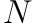.
2. Limited memory of size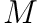.
3. Infinite disk space.
4. And read/write a consecutive block of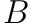between memory and disk.
5. Computation can only be done on cells in memory.

The cost is only the number of I/O’s operations (as a function of,and) so computations are free.

Even though the I/O model is still an oversimplification of the actual architecture it has shown to predict the asymptotic running time accurately in many cases.

We will consider how to obtain efficient sorting in the I/O model.

## 2 Sorting

One merge algorithm that can be adjusted to be efficient for the I/O model is the mergesort algorithm, specifically a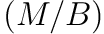-way mergesorting .

### 2.1 Merge

The objective is to merge a number of sorted arrays together. Say we want to keep one block for each array in memory at every given time then we can only merge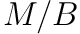sorted arrays if using a multi-array merge.

The-way merge proceed as follows. We merge the usual way by maintaining a pointer for each array starting at the first index. We then take the minimum value of the pointers and increment the pointer at the minimum value. The different is that if the pointer overflows the currently loaded block for the given array the next block in the array is loaded instead. Additionally, when the output in memory reaches a size ofit is written to disk and removed from memory.

##### Correctness

The argument for correctness is similar to normal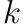-way merge but we also have to consider whether enough memory is available. Fortunately, we can keep allarray of sizein memory since they take up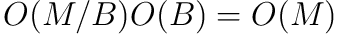in total.

##### I/O Complexity

Here we use that we have divided the input arrays and output array into chunks of size. The arrays are divided into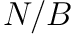blocks and the output is written usingblocks. So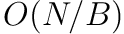I/O operations are spent.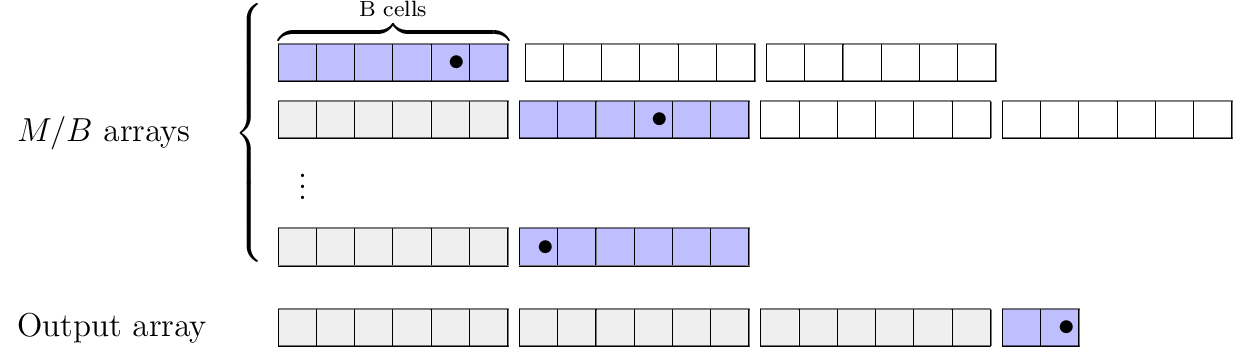Figure 2:-way merge.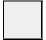indicates blocks that have already been in memory.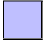indicates blocks that are currently in memory.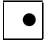denotes the current pointer for the array..

### 2.2 Sort

The merging step requires already sorted arrays. Observe that we can sortcells at a time efficiently since computations are free when the cells fit in memory.

So to turn an unsorted array of lengthinto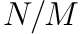sorted arrays (each of length) first load incells usingblocks and sort them using some sorting algorithm. Do this for allsub arrays.

##### I/O Complexity

Each sub array takesI/O reads and writes, and there areblock so number of I/O operations is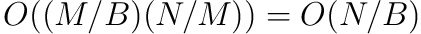.

### 2.3 Mergesort

Now, the idea is that we can combine the sorting and merging step to get an efficient mergesort in the I/O model.

Apply sec. 2.2 to getsorted arrays of length. Now, iteratively merge bundles ofarrays so that the number of arrays at each level is reduced by.

##### Complexity

To figure out the complexity for the entire computational tree (illustrated in fig. 3) we analyse how much each layer uses and how many layers we have.

How many I/O operations are used on each level? We count the level index from above. There are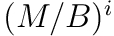arrays in total at level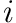each having size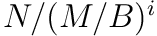thus taking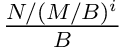operations to sort using sec. 2.1. In total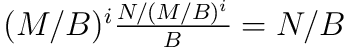.

How many levels are needed? The number of arrays at levelis. When this is equal towe can apply sec. 2.2. Solving foryields: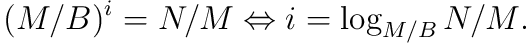So we spendfor each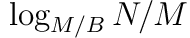levels and apply sec. 2.2 once on the final level taking. Number of I/O is thus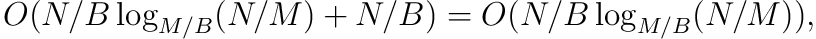which is actually a closer bound than what the article suggests.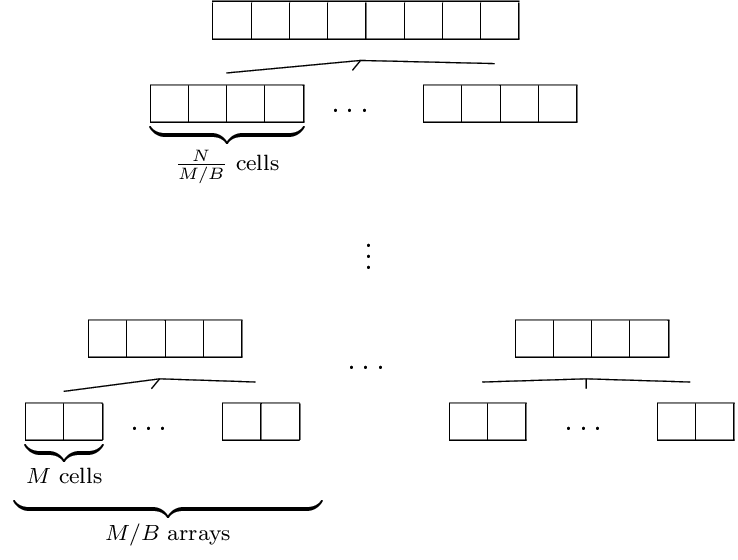Figure 3: Mergesort that sorts arrays in the bottom layer using sec. 2.2 and then merging bundles ofsorted arrays recursively until only one sorted array of lengthis left..

## 3 Conclusion

We have introduced the I/O model and shown an efficient mergesort algorithm when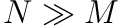. It has very similar running time as the standard mergesort with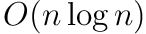but it differs by depending onand. Specifically it makes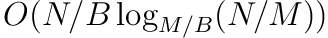I/O operations.

Next we will explore what we can do if we do not knowand.

 A. Aggarwal and S. Vitter Jeffrey, “The input/output complexity of sorting and related problems,” Commun. ACM, vol. 31, no. 9, pp. 1116–1127, Sep. 1988 [Online]. Available: http://doi.acm.org/10.1145/48529.48535